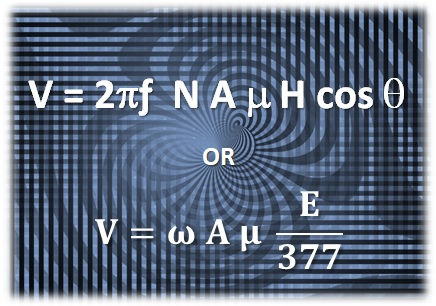Home » Radio » Shortwave Radio » Magnetic Loop Voltage – Inducing a Signal

# Magnetic Loop Voltage – Inducing a SignalWhether we are using an actual magnetic loop antenna or simulating an equivalent circuit, we need to understand how a signal voltage develops across the loop. Where does it come from?

Most of us live our lives without really understanding electromagnetic waves. Our ignorance is largely based on the inaccessibility of Maxwell’s equations which require some pretty advanced calculus. So, let’s put Maxwell aside and just work through the basic algebra of where a voltage across the terminals of a loop antenna comes from.

When a magnetic field impinges on a loop antenna, there is a time varying magnetic flux, cutting through the conductor. The flux density, B, is just a bunch of magnetic flux lines cutting through a specific area. The basic formula is B=μH where μ is permeability and H is the magnetizing force, in this case a radio wave.

Permeability, μ is how we measure the ability of a material to support the formation of a magnetic field within itself. The permeability of my loop’s aluminum material is 1.26 x 10-6 Henries/meter. The magnetizing force is the magnetic field strength, in amps/meter of the radio wave. You can translate between the electric and magnetic field strengths using the impedance of free space, or 377 Ω.

The basic formula for voltage created at the output of an open circuit loop is:

V = ω N A μMAT H cosθ

This tells us the voltage increases with frequency (ω=2πƒ ), azimuth of signal (θ) and permeability of loop material (μ). It also increases with loop area (A) and number of turns (N). Finally, the magnetic field strength created is equal to the electric field strength divided by wave impedance (H= E ÷ 377).

Since we have a one turn loop (N=1) and can assume maximum azimuth (cos θ= 1), we can simplify the voltage formula as follows:

V = ω A  μ (E ÷ 377)

## Magnetic Loop Voltage Sample Calculation

So, let’s start with a typical weak radio signal passing through a 1 meter diameter loop antenna, say an electric field of 1 μV/m at 1 MHz. This will create a voltage across the loop terminals of 16 nV, as follows:

V = 2 • π • (1·106 ) • (π·0.52) • (1.26·10-6) • (1·10-6 ÷ 377) = 16 nano-volts.

Clearly, magnetic fields create very weak voltages in a loop antenna. Even a relatively strong electric field of S9 or 50μV will only induce a one micro-volt signal in the antenna from the magnetic field.

Getting the signal strength up to usable levels at the receiver requires gain, much of which you can accomplish with a high Q tuned loop and a modest amplifier. But an un-tuned or wideband loop is a different story.

This site uses Akismet to reduce spam. Learn how your comment data is processed.